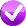# Building Java Programs, 3rd edition

## Lab: Cumulative Algorithms

Except where otherwise noted, the contents of this document are Copyright 2013 Stuart Reges and Marty Stepp.

lab document created by Marty Stepp, Stuart Reges and Whitaker Brand

# Lab goals

Goals for this problem set:

• write cumulative algorithms that compute their data over time
• Where you see this icon, you can click it to check the problem in Practice-It!# Cumulative algorithms

• A cumulative algorithm is one where you incrementally accumulate a larger value by repeatedly adding, multiplying, etc., and storing the result into a variable over and over.
• Key aspect of a cumulative algorithm: A loop, and a variable declared outside the loop whose value is modified inside the loop.
• Example: Cumulative algorithm to sum the numbers 1-100:
```int sum = 0;
for (int i = 1; i <= 100; i++) {
sum = sum + i;
}
System.out.println(sum);    // 5050
```
• Some of the following problems ask you to write cumulative algorithms.

# Exercise : `Scanner` sum

Copy and paste the following code into jGrasp.

```public class SumNumbers {
public static void main(String[] args) {
int low = 1;
int high = 1000;
int sum = 0;
for (int i = low; i <= high; i++) {
sum += i;
}
System.out.println("sum = " + sum);
}
}
```

continued on next slide...

# Exercise : `Scanner` sum

Modify the code to use a `Scanner` to prompt the user for the values of `low` and `high`. Below is a sample execution in which the user asks for the same values as in the original program (1 through 1000):

```low? 1
high? 1000
sum = 500500
```

Below is an execution with different values for `low` and `high`:

```low? 300
high? 5297
sum = 13986903
```

You should exactly reproduce this format.

Use the Output Comparison Tool to check your work.

# Exercise : powWrite a method named `pow` that accepts a base and an exponent as parameters and returns the base raised to the given power. For example, the call `pow(3, 4)` returns 3 * 3 * 3 * 3 or 81. Do not use `Math.pow` in your solution; use a cumulative algorithm instead. Assume that the base and exponent are non-negative.

# Exercise : repl• Write a method named `repl` that accepts a `String` and a number of repetitions as parameters and returns the `String` concatenated that many times. For example, the call `repl("hello", 3)` returns `"hellohellohello"`. If the number of repetitions is 0 or less, an empty string is returned.
• Try solving this problem in Practice-It! using the link above.
• (Hint: This is best solved with a cumulative algorithm. Start with an empty string and build it up piece by piece.)

# Exercise : longestNameWrite a method named `longestName` that reads names typed by the user and prints the longest name (the name that contains the most characters) in the format shown below. Your method should accept a console `Scanner` and an integer n as parameters and should then prompt for n names.

A sample execution of the call `longestName(console, 4)` might look like the following:

```name #1? roy
name #2? DANE
name #3? sTeFaNiE
name #4? Erik
Stefanie's name is longest
```

Try to solve this problem in Practice-It: click on the check-mark above!